# Matplotlib 101: Learn Matplotlib in 10 minutesIn this blog post, I will show you how to use matplotlib effectively and efficiently. You will learn how to create basic plots, customise them with different styles and features, and save them for later use. By the end of this post, you will be able to create beautiful and informative graphs with just a few lines of code.

## Installing Matplotlib

There are two ways to install matplotlib:

### Using pip

``````pip install matplotlib
``````

### Using conda

``````conda install -c conda-forge matplotlib
``````

## Importing Matplotlib

Import matplotlib for plotting and visualisation and import numpy to use some mathematical operations and functions.

Just to make our visualization look nicer, we won’t use the default plot style, we’ll use the seaborn style for our plotting.

``````import matplotlib.pyplot as plt
import numpy as np

plt.style.use(['seaborn'])
``````

Now, let’s jump into plotting!!!

## Line Plot

### Simple Plot

Let’s first generate dummy data to plot.

``````x = np.linspace(0,15,30)
y = np.sin(x)
``````

Here, `x` is a numpy array having numbers from 0 to 15 and `y` is a sine function of `x`.

Now, it’s the time that you are waiting for, PLOTTING!!!

``````plt.plot(x, y)
``````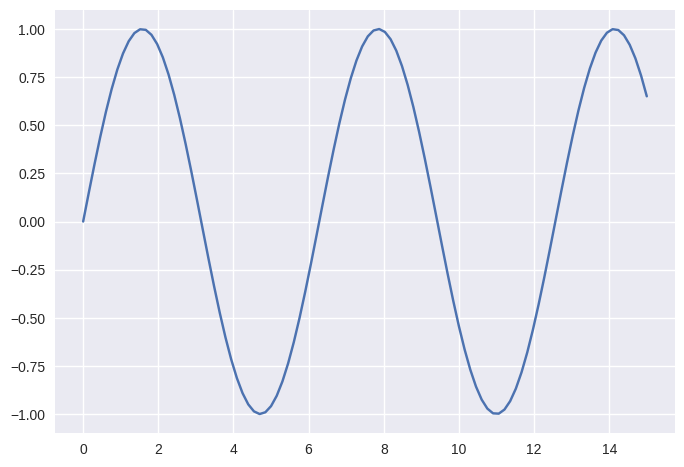Now, how about changing the line to something else like only dashes or only dots or dots and dashes?

The third parameter is used to set the line style whether it be dashes or dots.

### Only Dashes

``````# -- is used for only dashes
plt.plot(x,y, '--')
``````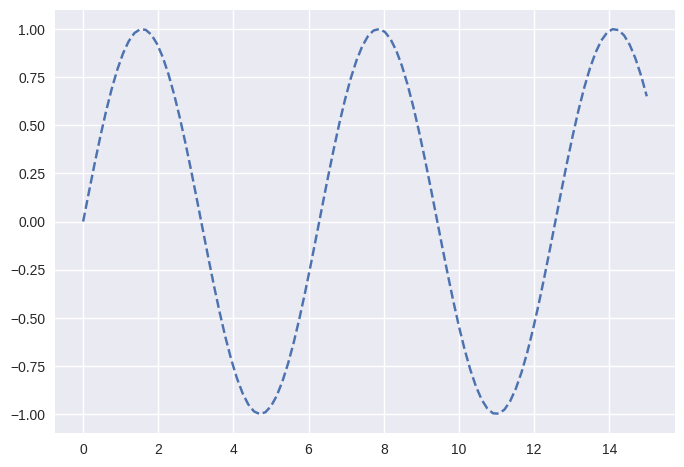### Only Dots

``````# 'o' is used for only dots
plt.plot(x, y, 'o')
``````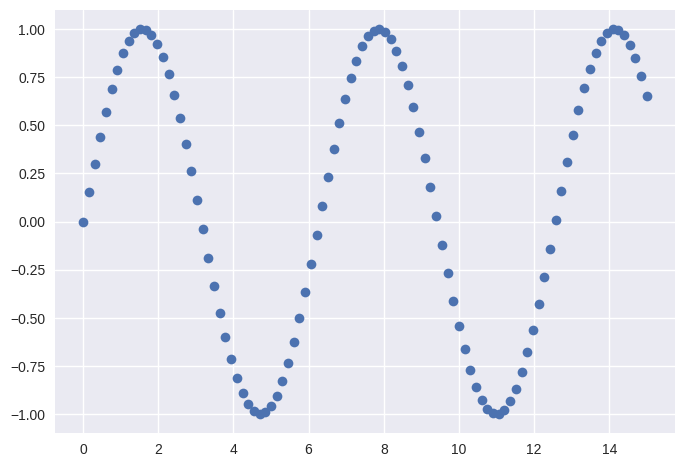### Dashes and Dots

``````# '--o' is used for dashes and dots
plt.plot(x,y, '--o')
``````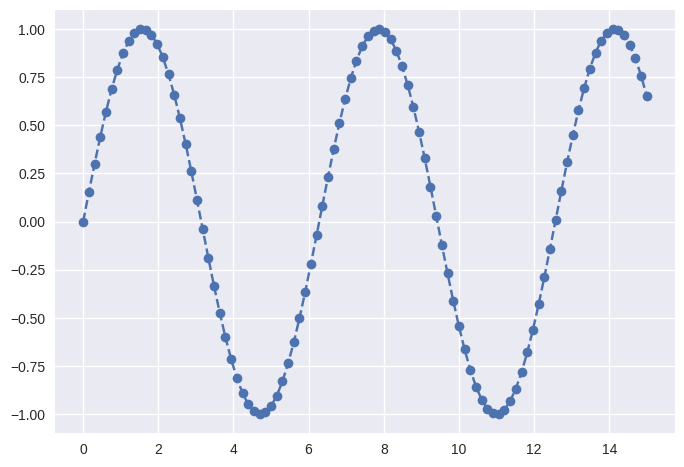You can change the colour of the line and you can also change the width of the line.

``````# `color` is used to change the colour of your line
# `lw` stands for line-width and this is used to set the width of the line
plt.plot(x,y, color='teal', lw=2)
``````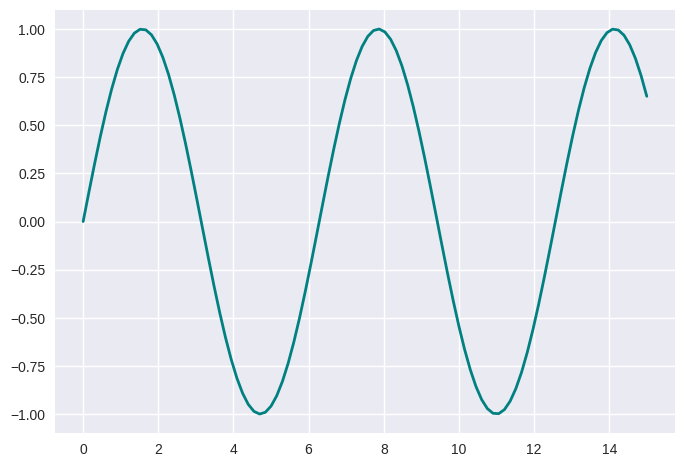If you are using the `dashes and dots` kind of line, then you can also change the size of the dot using the `ms` parameter. Here `ms` stands for marker size.

``````plt.plot(x,y, '--o', color='teal', lw=.5, ms=5)
``````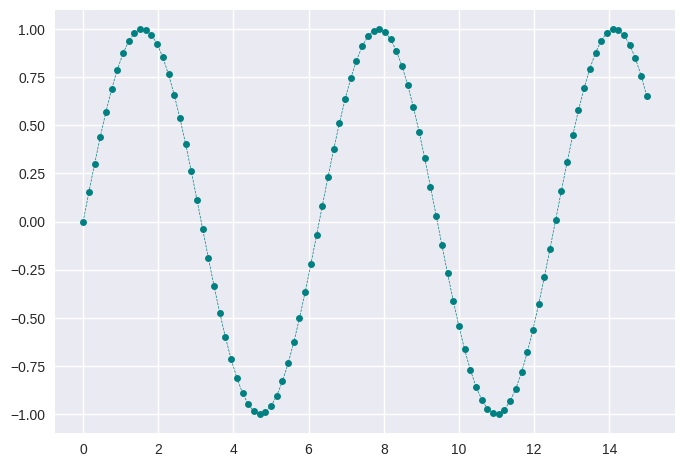You can take a look in the matplotlib documentation for the full list of colours — Click Here

## Figure

Now, let's say you don't want this kind of square figure, you want to have a figure which resembles a rectangle shape, then you can set the size of the figure by using the `figure()`method.

In `figsize` you should mention the units on the x-axis and y-axis respectively and it’ll set the figure size accordingly.

``````plt.figure(figsize=(12,3))
plt.plot(x,y, '-', color='teal', lw=2)
``````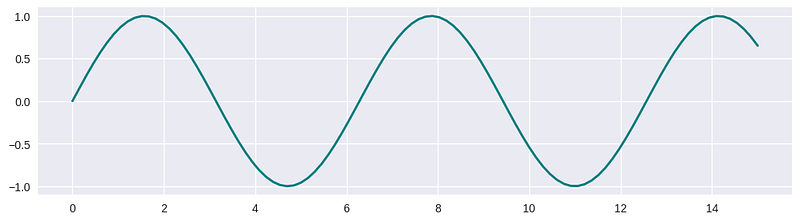Okay, I think we have learnt quite a lot, but something is missing.

As you know, a figure should contain labels on the x-axis and y-axis and there should be a title for the figure. So, let's add these things to the figure.

``````# setting the figure size
plt.figure(figsize=(12,3))

# plotting the line
plt.plot(x,y, 'o--', color='teal', lw=2, ms=10)

# setting the label of the x-axis and also changing the font size to 16
plt.xlabel('x', fontsize=16)

# setting the label of the y-axis
plt.ylabel('sin(x)')

# setting the title of the figure
plt.title('Sine Wave')
``````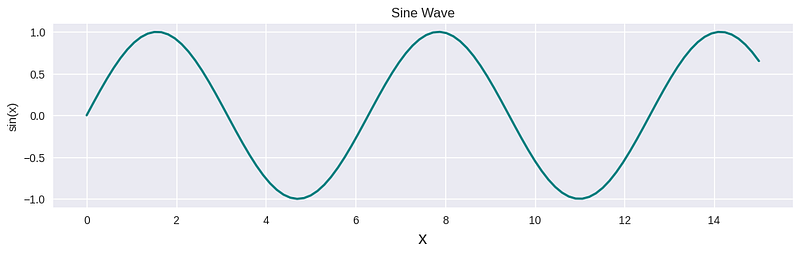### Multiple Plot

Let's say you want to compare the sine and cosine waves and to do that you want to put these curves in the same figure.

First, let’s create x and y values for the cosine wave.

``````x2 = np.linspace(0, 15, 100)
y2 = np.cos(x2)
``````

Now, let’s plot for the sine and cosine waves in the same figure.

``````# to set the figure size
plt.figure(figsize=(8,3))

# to plot the sine wave using x and y
plt.plot(x,y, '-')

# to plot the cosine wave using x2 and y2
plt.plot(x2,y2)

# setting the x-axis label of the figure
plt.xlabel('x', fontsize=16)

# setting the y-axis label of the figure
plt.ylabel('Wave')

# setting the title of the figure
plt.title('Sine and Cosine Wave')
``````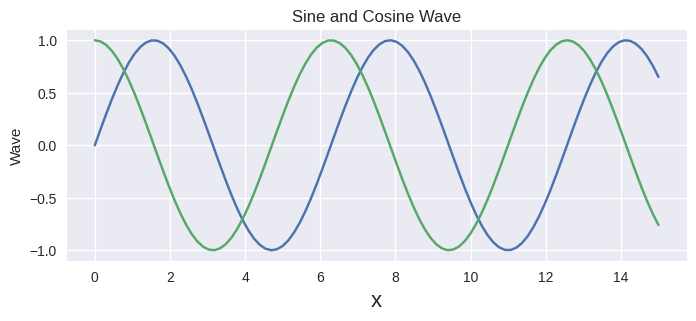The above figure looks nice and clean, but there is one problem.

If you don’t know how the sine and cosine wave looks like, then there is no way to recognise which curve is a sine wave and which one is a cosine wave.

To solve this issue we have Legend.

So, let’s add a legend to our figure.

``````# to set the figure size
plt.figure(figsize=(8,3))

# to plot the sine wave using x and y
plt.plot(x,y, '-', label='Sine')

# to plot the cosine wave using x2 and y2
plt.plot(x2,y2, label='Cosine')

# setting the x-axis label of the figure
plt.xlabel('x', fontsize=16)

# setting the y-axis label of the figure
plt.ylabel('Wave')

# setting the title of the figure
plt.title('Sine and Cosine Wave')

# setting the font size to 12 and the location is set to best
plt.legend(loc='best', fontsize=12)
``````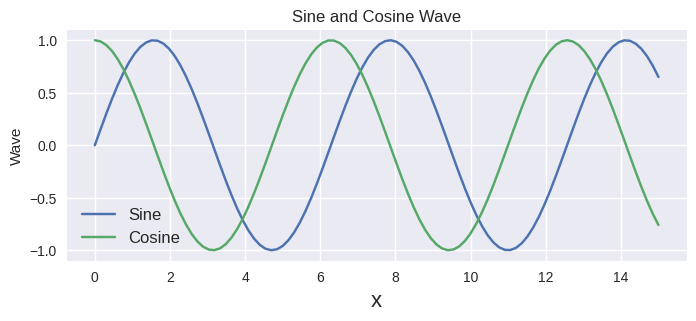Notice, in the above code the `plot()` function also has another parameter `label` which is used to let the legend know the label of the plot.

In the above code, `legend` has two parameters, `loc` and `fontsize`.

• `loc` is used to set the location of the legend in the figure. I’ve asked the function to use the `best` location but there are multiple options that you can choose. Check out the documentation to learn more about the options — Click Here

• `fontsize` is used to set the font size of the content inside the legend.

With this I think, we have covered a good amount of things regarding line graphs. Now, let’s head on to different graphs, starting with a bar graph.

### Bar Graphs

Let’s think of a scenario. In your school or college, there are 100 students and you asked them to choose their favourite programming language.

70 of them said Python, 20 of them said C++ and 10 of them said Java.

Now, to visualise this you can use bar graphs.

``````programming_languages = ['Python', 'C++', 'Java']
students_choice = [70, 20, 10]
plt.bar(programming_languages, students_choice)
``````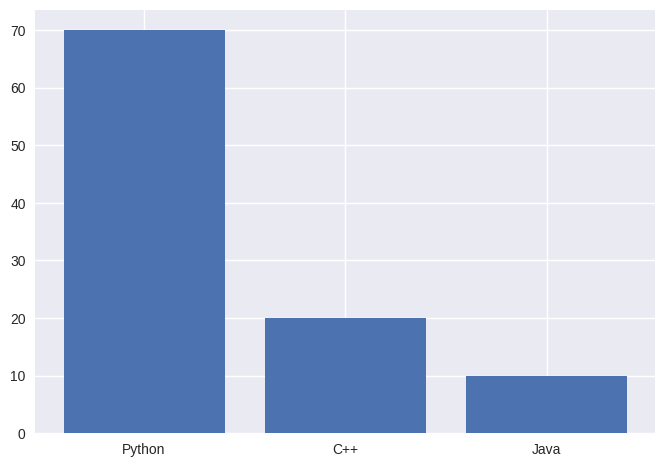The above bar graph looks decent but let’s customise this to make it look nicer.

``````bar_plot = plt.bar(programming_languages, students_choice)
bar_plot.set_hatch('/')
bar_plot.set_hatch('\\')
bar_plot.set_hatch('/\\')
plt.figure(figsize=(12,8))
plt.show()
``````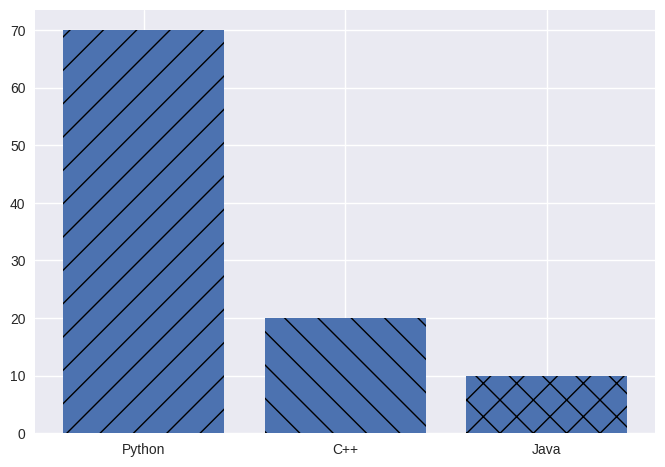This is much better, I love these designs. By using `set_hatch`, you can add the patterns to your graph. To learn about other hatch patterns, check out the documentation — Click Here

From now on, we’ll be using the Titanic Dataset which we used in the PANDAS 101 post. Click here to Download the Dataset

### Histogram

Let's say you want to know how many passengers have ages from 0–10, how many have ages from 10–20 and so on. Then you can use a histogram.

``````# we need to know the count for every 10 range interval
bins = [0,10,20,30,40,50,60,70,80,90,100]

# hist() takes a column as data and number of bins
plt.hist(df["Age"], bins=bins)

# use xticks to set the x-axis values
plt.xticks(bins)
plt.xlabel("Age")
plt.ylabel("Number of Passengers")
plt.title("Number of passengers within a interval")
plt.show()
``````We first set bins which act as an interval of 10. So, the first bin will be from 0–10, then the second bin will be from 10–20 and so on.

Now, just plot the histogram using the “Age” column and the bins.

I have set the `xticks` to bins, so that it’ll be easy to visualize the bins.

We can also change the colour as we did with other graphs.

``````#change colour
bins = [0,10,20,30,40,50,60,70,80,90,100]
plt.hist(df["Age"], bins=bins, color='teal')
plt.xticks(bins)
plt.xlabel("Age")
plt.ylabel("Number of Passengers")
plt.title("Number of passengers within a interval")
plt.show()
``````### Pie Chart

Let's say you want to check out of all the male passengers, how many of them survived and out of all female passengers, how many of them survived.

``````# getting the count of male passengers
male = df.loc[df["Sex"] == "male"].count()

# getting the count of male and survived passengers
survived_male = df.loc[(df["Sex"] == "male") & (df["Survived"] == 1)].count()

# getting the count of female passengers
female = df.loc[df["Sex"] == "female"].count()

# getting the count of female and survived passengers
survived_female = df.loc[(df["Sex"] == "female") & (df["Survived"] == 1)].count()

# setting labels for each of them
labels = ['male', 'female']

# setting colours
colors = ['orange','teal']

# plotting the pie chart using the above data
plt.pie([survived_male, survived_female], labels=labels, colors=colors, hatch=["/","o"])
plt.title('male vs female survivors')
plt.legend(loc="upper left", fontsize=12)
plt.show()
``````You may be wondering why `count()`.

Because, `count()` will return a data frame and our focus is to get the number of passengers, so if we only choose the first column of that data frame i.e `PassengerId` then our job will be done.

You can use hatch here too.

The rest of the things are pretty much similar to the other graphs i.e, you can set the title, colour, labels and legend of the graph.

Note: If you are not able to understand how we got the male and survived passengers data or female and survived passengers data, then you should learn about pandas first, and I have a great post on it. Check it out Here

### Subplots

Let's say you want to have a figure which consists of many different figures(not talking about multiple plots), then you can use `subplots()` to do that.

Subplots are useful when we want to display multiple plots in a single figure, such as comparing different datasets or showing different aspects of the same data.

To create subplots in matplotlib, we can use the pyplot.subplots() function, which returns a figure object and an array of axes objects. The figure object represents the entire figure, and the axes objects represent the individual plots within the figure. We can specify the number of rows and columns of the subplot grid as arguments to the subplots() function, and optionally pass other parameters to control the appearance and behaviour of the subplots.

For example, let’s create a 2x2 grid of subplots, and plot some sine and cosine curves on them:

``````
import matplotlib.pyplot as plt
import numpy as np
# Create some sample data
x = np.linspace(0, 2*np.pi, 100)
y1 = np.sin(x)
y2 = np.cos(x)
y3 = np.sin(x)**2
y4 = np.cos(x)**2
# Create a figure and a 2x2 grid of subplots
fig, axs = plt.subplots(2, 2)
# Plot the data on each subplot
axs[0, 0].plot(x, y1, color='blue')
axs[0, 1].plot(x, y2, color='orange')
axs[1, 0].plot(x, y3, color='green')
axs[1, 1].plot(x, y4, color='red')
# Add some titles and labels
fig.suptitle('Matplotlib Subplots Example')
axs[0, 0].set_title('Sine')
axs[0, 1].set_title('Cosine')
axs[1, 0].set_title('Sine Squared')
axs[1, 1].set_title('Cosine Squared')
for ax in axs.flat:
ax.set_xlabel('x')
ax.set_ylabel('y')
# Adjust the spacing between subplots
plt.tight_layout()
# Show the figure
plt.show()
``````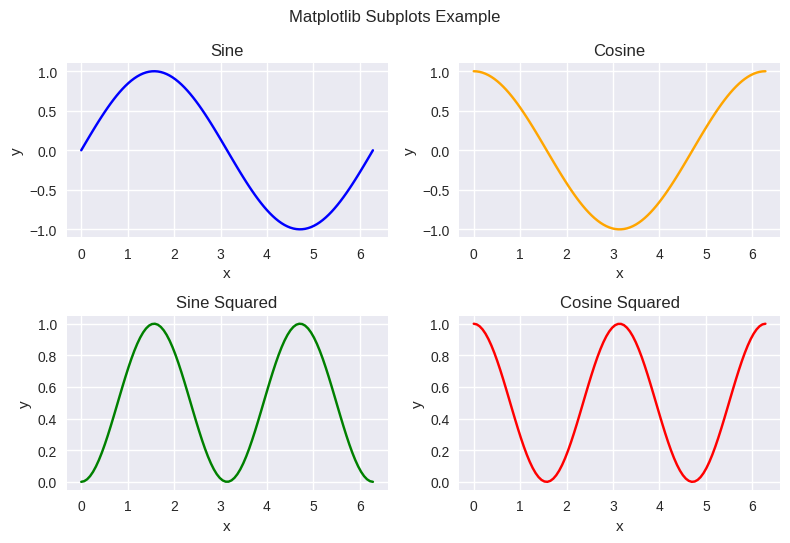• `subplots` have two parameters: `number_of_rows` and `number_of_columns` and this will make a grid of the specified number of rows and columns.

• Everything remains the same except, here you don’t need to use `plt.plot` instead you will use `axs[row_position, column_position]`. This will use the specific row and column position for the plot.

• Here, to set the x and y label of each figure, you need to use `set_xlabel()` and `set_ylabel()` respectively.

As you can see, we have created four subplots in a single figure, each with its title and labels.

There are many other parameters and methods that we can use to customize our subplots. For more details and examples, you can refer to the official documentation — Click Here

### Conclusion

In this blog post, we have learned about matplotlib, a powerful Python library for creating and customising various types of plots and charts. We have seen how to import matplotlib, create various charts and plots, add labels and titles, and adjust colours and styles.

Matplotlib is a versatile and flexible tool that can help us visualise and communicate our data effectively. I hope you have enjoyed this article on Matplotlib and feel inspired to create your plots and charts using this library.